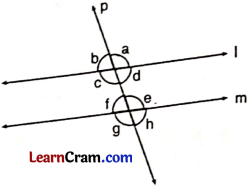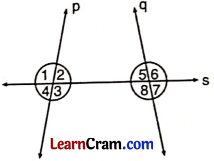# DAV Class 6 Maths Chapter 11 Worksheet 2 Solutions

The DAV Maths Book Class 6 Solutions and DAV Class 6 Maths Chapter 11 Worksheet 2 Solutions of Transversal and Pairs of Lines offer comprehensive answers to textbook questions.

## DAV Class 6 Maths Ch 11 WS 2 Solutions

Question 1.
In the given figure, lines l and m are intersected by a transversal ‘p’. Identify the following:(a) alternate angles of ∠a and ∠c
(b) corresponding angles of ∠b and ∠d
(c) all the interior angles
(d) all the exterior angles
(a) Alternate angle of ∠a is ∠g and for ∠c it is ∠e.
(b) Corresponding angles of ∠b and ∠d are ∠f and ∠h respectively.
(c) Interior angles are ∠c, ∠d, ∠f and ∠e.
(d) Exterior angles are ∠a, ∠b, ∠g and ∠h.

Question 2.
In the given figure, lines p and q are intersected by a transversal s. Find the pairs of:(a) corresponding angles
(b) alternate interior angles